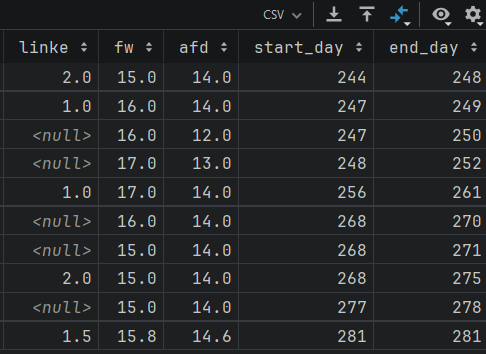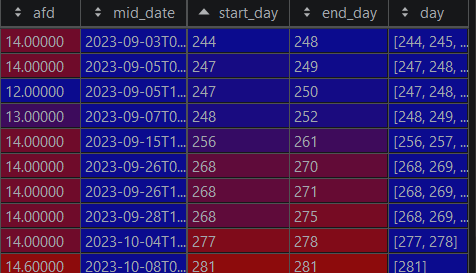# Explorer: DataFrame with StartDate and EndDate columns to timeline

I have a DataFrame Value columns and a Column with a start date (NaiveDateTime) and an end date (NaiveDateTime) at which the values where meassured. I would like to “unroll” the dateframe so that, I get a row for every date (day of the year), for every sample in the data frame.

I know, how I would do it in pandas (perhaps not the most elegant solution).
I would compute the day of the year (day) of the start and end dates, construct a list of every doy withing this range as a new column and than explode to return t 1st NF and that it is.

How would I do that with Explorer?

I started with:

``````filtered_polls = polls
|> DF.filter(start_date >= ^start_date)

polls_timeline = filtered_polls
|> DF.put("start_day", S.day_of_year(filtered_polls["start_date"]))
|> DF.put("end_day", S.day_of_year(filtered_polls["end_date"]))

polls_timeline
|> DF.put("date_range", polls_timeline["start_day"]..polls_timeline["end_day"])
|> DF.print()
``````

Which is due to the range can not work on two series. But un two zipped lists.

``````dates = polls_timeline["start_day"]
|> S.to_list()
|> Enum.zip(S.to_list(polls_timeline["end_day"]))
|> Enum.map(fn {start_date, end_date} -> start_date..end_date end)
|> Enum.map(&Enum.to_list(&1))

polls_timeline
|> DF.put("dates", dates)
|> DF.print()
``````

Which does not work. Because I can not add a list as data type. Next I still would not know how to unroll.

What happens if you call S.from_list() before? Something like:

``````dates = polls_timeline["start_day"]
|> S.to_list()
|> Enum.zip(S.to_list(polls_timeline["end_day"]))
|> Enum.flat_map(fn {start_date, end_date} -> start_date..end_date end)
|> S.from_list()
``````

a flatmap is really able to work with a series. But this still cannot be put, as the lengths do not match.

At the start I have a dataframe like:

| start_date | end_date | value1 | value2 |
| <datetime[μs]> |<datetime[μs]> | | |
+===========+==============+=====================+
| 2023-08-29 16:30:13.000000 | 2023-08-30 16:30:13.000000| 0.2758| 0.30290 |

and want that every row unrolls to something like:

| day | value1 | value2 |
| | | |
+===========+==============+=====================+
| 241 | 0.2758| 0.30290 |
±----------±---------------------------±--------------------+
| 242 | 0.2758| 0.30290 |

After the flatmap have literaly gotten the day column correct, but the value columns still have the original length. Therefore I can not put the new col.

P.S.: The next steps would be, group(day)->mean(values)-> distinct(). I know how to that. The final step is, what I do not know. How would I fill all the missing day between the first and the last date, that are missing and fill the value columns with nils, so that I cann fill the value will last values. Although I would like to fill with linear interpolation. Still don’t know how to do that.
In the end I would have a timeline with all values filled and a row for each day.^^

Hi,
I’m not really sure I understand the context, in case it helps?
polls_timeline =
polls_timeline
|> DF.map_rows(fn row →
start_day = elem(row, 2) # Assume than start_day at position 2
end_day = elem(row, 3) # Assume end_day at position 3
date_range = Enum.to_list(start_day…end_day)
Tuple.append(row, date_range)
end)

new_rows =
polls_timeline
|> DF.to_list()
|> Enum.flat_map(fn row →
date_range = List.last(row)
Enum.map(date_range, fn date →
List.replace_at(row, -1, date)
end)
end)

new_df = DF.new(new_rows, names: DF.names(polls_timeline))

@nseaSeb map_rows was neither included in the Documentation of Explorer, nor could I use it in the git Version I currently use. Could you tell, me a bit more about it?

Yes, I’m not at all sure about this. The map_rows/2 function is not available in Elixir’s Explorer library. It seems to be a function of the df library in R.

However, you can get a similar effect by using Enum.map/2 to iterate over each row of the DataFrame, unless I’m mistaken?

Okay, so something like pandas iterrows or apply. I assume that is not possible in Elixir to Enum.map over a Data Frame.

The closest I have seen is the pivot_longer function. But it’s works on existing columns that are combined into one column. But instead of columns I have a list of data (of variable length).
@josevalim I hope that makes clear, what I want. Do you know how to address it?

Hey @sehHeiden,

I wasn’t able to follow why @josevalim’s suggestion doesn’t work. You say:

I think you’ll need to do the grouping/etc. while it’s still a list. Then I think the lengths should match.

Also, if you’re trying to do interpolation with dates, this answer might help.

@billylanchantin I musst be afk and try clear the problem from remote as good as I can.

What I meant is, the original DataFrame has about ten entries.
Than I take the dates, as start and End number and construct either a list of list, or what @josevalim proposed A flat_map which creates a flat list list of length 40 or so and than makes a Series out of it.
So the problems from my point of few are:
a) Putting a Series of length 40 into a DataFrame of Shape 10, n_cols is not possible?!
b) I don’t know to transform the DataFrame to a length shape of 40, n_cols and preserve the same row order as in the Series.
You talked about grouping. How would it help in this topic?

I’m taking some liberties to illustrate the idea.

``````alias Explorer.Series, as: S
require Explorer.DataFrame, as: DF

data = [
%{start_date: ~D[2023-01-01], end_date: ~D[2023-01-03], value: 1},
%{start_date: ~D[2023-02-01], end_date: ~D[2023-02-03], value: 2}
]

df =
data
|> DF.new()
|> DF.mutate(
start_day: day_of_year(start_date),
end_day: day_of_year(end_date)
)

computed_values =
df
|> DF.to_rows()
|> Enum.flat_map(fn row ->
Enum.map(row["start_day"]..row["end_day"], fn day ->
# Hold onto "start_day" so we group-then-sort by it later
row |> Map.take(["start_day", "value"]) |> Map.put("day", day)
end)
end)
# Do whatever computation you're trying to do here.
|> Enum.map(fn %{"day" => day, "value" => value} = row ->
Map.put(row, "value2", day + value)
end)
# Now we can group by "start_day", which makes n_rows match again.
|> Enum.group_by(&(&1["start_day"]), &(&1["value2"]))
|> Enum.sort()
|> Enum.map(fn {_start_day, values} -> Enum.sum(values) end)

DF.put(df, :computed_values, computed_values)
``````

A few points:

1. The above is not optimal. I focused in individual steps to clarify the idea.
2. I admit, I don’t quite know what unrolling the list accomplishes if you need to collapse it back down. Presumably, for most computations you could skip the unrolling and just operate on the dataframe directly.

If you show exactly what computation you’re trying to accomplish, I could say more. But the above is what I meant by “grouping/etc”.

@billylanchantin I did it in Python Pandas and ilustrate it with some images.
I start with a DataFrame with start and Enddates and some values:Than I add a column (last column), that holds the days from start to end date:You see the brackets. Which implies the list. Than I “explode” the DataFrame. So that from any value in the list, the other row values are copied and the row-exists n times.

The image shows that line zero exists now 5 times, as the list in column `day` is exploded.

Here is the python code:

``````from pandas import read_csv

data_df["day"] = data_df.apply(lambda x: list(range(x["start_day"], x["end_day"]+1)), axis="columns")
data_df = data_df.explode("day")
data_df.to_csv("doy_data_unrolled.csv", index=False)
``````

How would I reach the result in Explorer?

@billylanchantin I just checked Polars also can do that. Polars Explode

But it’s not in the explorer documentation, olso the types list and array…
Am I right?

Thank you! That makes it much easier to answer.

So I think @josevalim’s answer was what you want (for now, see below):

``````df = DF.new([
%{start_day: 244, end_day: 248, fw: 15.0},
%{start_day: 247, end_day: 249, fw: 16.0}
])

df
|> DF.to_rows()
|> Enum.with_index()
|> Enum.flat_map(fn {row, index} ->
row["start_day"]..row["end_day"]
|> Enum.map(&Map.merge(row, %{"day" => &1, "index" => index}))
end)
|> DF.new()
|> DF.arrange(index) # You can order by any column
|> DF.print(limit: :infinity)
# +---------------------------------------------------------+
# |        Explorer DataFrame: [rows: 8, columns: 5]        |
# +-----------+-----------+---------+-----------+-----------+
# |    day    |  end_day  |   fw    |   index   | start_day |
# | <integer> | <integer> | <float> | <integer> | <integer> |
# +===========+===========+=========+===========+===========+
# | 244       | 248       | 15.0    | 0         | 244       |
# +-----------+-----------+---------+-----------+-----------+
# | 245       | 248       | 15.0    | 0         | 244       |
# +-----------+-----------+---------+-----------+-----------+
# | 246       | 248       | 15.0    | 0         | 244       |
# +-----------+-----------+---------+-----------+-----------+
# | 247       | 248       | 15.0    | 0         | 244       |
# +-----------+-----------+---------+-----------+-----------+
# | 248       | 248       | 15.0    | 0         | 244       |
# +-----------+-----------+---------+-----------+-----------+
# | 247       | 249       | 16.0    | 1         | 247       |
# +-----------+-----------+---------+-----------+-----------+
# | 248       | 249       | 16.0    | 1         | 247       |
# +-----------+-----------+---------+-----------+-----------+
# | 249       | 249       | 16.0    | 1         | 247       |
# +-----------+-----------+---------+-----------+-----------+
``````

Also, I don’t want to speak for the core team, but `explode` does appear to be on the roadmap:

So if/when that functionality is added, you can do a nearly one-to-one translation of the Pandas code.

Thanks for you help. You completly deconstructed it computed the inner list and than made a new DataFrame.

I think, we don’t have to do everything, like others do, but it has to make sense within its inner logic.